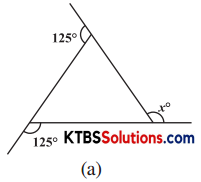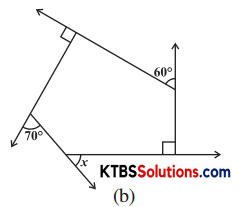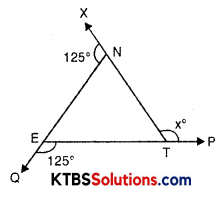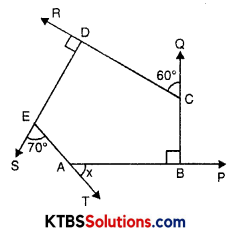# KSEEB Solutions for Class 8 Maths Chapter 3 Understanding Quadrilaterals Ex 3.2

You can Download KSEEB Solutions for Class 8 Maths Chapter 3 Understanding Quadrilaterals Ex 3.2 Questions and Answers helps you to revise the complete syllabus.

## KSEEB Solutions for Class 8 Maths Chapter 3 Understanding Quadrilaterals Ex 3.2

Question 1.
Find x in the following figures:Solution:∠XNE + ∠ENT = 180° (Linear pair)
⇒ 125° + ∠ENT = 180°
⇒ ∠ENT = 180° – 125°
∴ ∠ENT = 55°
Similarly, ∠NET = 55°
In ∆NET,
∠N + ∠E + ∠T = 180°
⇒ 55° + 55° + ∠T = 180°
⇒ 110° + ∠T = 180°
⇒ ∠T = 180° – 110°
∴ ∠T = 70°
∠NTE + ∠x° = 180°
⇒ 70° + ∠x = 180°
⇒ ∠x = 180° – 70°
∴ ∠x = 110°(b) ∠DCQ + ∠DCB = 180° (Linear pair)
⇒ 60° + ∠DCB = 180°
⇒ ∠DCB = 180° – 60°
∴ ∠DCB = 120°
∠AES + ∠AED = 180° (Linear pair)
⇒ 70° + ∠AED = 180°
⇒ ∠AED = 180° – 70°
∴ ∠AED = 110°
∠RDE + ∠EDC = 180° (Linear pair)
⇒ 90° + ∠EDC = 180°
⇒ ∠EDC = 180° – 90°
∴ ∠EDC = 90°
∠ABC + ∠CBP = 180° (Linear pair)
⇒ 90° + ∠CBP = 180°
⇒ ∠CBP = 180° – 90°
∴ ∠CBP = 90°
∠SEA + ∠TAB + ∠CBP + ∠DCQ + ∠RDE = 360° (Sum of exterior angles of a polygon)
⇒ 70° + x + 90° + 60° + 90° = 360°
⇒ x + 310° = 360°
⇒ ∠x = 360° – 310°
∴ ∠x = 50°Question 2.
Find the measure of each exterior angle of a regular polygon of
(i) 9 sides
(ii) 15 sides.
Solution:
(i) Sum of exterior angles = 360°
No. of sides = 9
∴ Measure of each exterior angle = $$\frac{360^{\circ}}{9}$$ = 40°

(ii) Sum of exterior angles = 360°
No. of sides = 15
∴ Measure of each exterior angle = $$\frac{360^{\circ}}{15}$$ = 24°

Question 3.
How many sides does a regular polygon have if the measure of an exterior angle is 24°?
Solution:
Sum of measures of exterior angles of a polygon = 360°
Measure of exterior angle = 24°
∴ No. of sides = $$\frac{360^{\circ}}{24}$$ = 15
Hence, No. of sides of a polygon = 15Question 4.
How many sides does a regular polygon have if each of its interior angles is 165°?
Solution:
Each interior angle = 165°
Each exterior angle = 180° – 165° = 15°
Sum of measure of exterior angles = 360°
No. of sides = $$\frac{360^{\circ}}{15}$$ = 24
Hence, polygon has 24 sides.

Question 5.
(a) Is it possible to have a regular polygon with measure of each exterior angle as 22°?
(b) Can it be an interior angle of a regular polygon? Why?
Solution:
(a) No. (Since, 22 is not a divisor of 360°.)
(b) No, because each exterior angle is 180° – 22° = 158° which is not a divisor of 360°.Question 6.
(a) What is the minimum interior angle possible for a regular polygon? Why?
(b) What is the maximum exterior angle possible for a regular polygon?
Solution:
(a) Equilateral triangle is a regular polygon of 3 sides.
So, each interior angle = $$\frac{180^{\circ}}{3}$$ = 60°

(b) By (a) we can see that the greatest exterior angle is (180° – 120°) = 60°